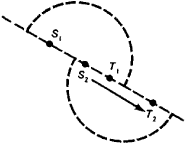# heliometer

(redirected from heliometry)
Also found in: Dictionary, Thesaurus.
Related to heliometry: telemetry

## heliometer

(hee-lee-om -ĕ-ter) An obsolete refracting telescope with a split objective lens that was used to measure the angular distance between two stars and to measure position angles. The two sections of the objective were moved until the images of the stars produced in the two sections were made to coincide. The amount of movement gave a precise measure of the angular distance.
Collins Dictionary of Astronomy © Market House Books Ltd, 2006
The following article is from The Great Soviet Encyclopedia (1979). It might be outdated or ideologically biased.

## Heliometer

an astrometric instrument for measuring small angles (up to 1°) on the celestial sphere. The idea for a heliometer was suggested by the Danish astronomer O. Römer in 1675, and the final design was realized by the British optician J. Dollond in 1753. Originally the heliometer was used to measure the diameter of the sun, hence its name. Later, it was used to measure the diameters of the moon and planets and the planetocentric coordinates of the orbits of the satellites of the planets, as well as to measure binary stars and to determine the parallaxes of stars. It consists of a refractor, the objective of which is cut along a diameter. The halves of the objective can be moved along the cut with the aid of a micrometer screw. As this is done, the image of the celestial object in the focal plane of the objective splits, and both halves are displaced with respect to each other. By matching the opposing points of a celestial object’s diameter, the images of a binary star’s components, and so forth, and measuring the mutual displacement of the halves of the objective, it is possible to compute the angular distance between the matched points. (In Figure 1 the images of the left star S2 and of the right star T1 are being matched.) In order to set the direction of displacement of the halves of the objective parallel to the segment joining both points, the objective part can be turned. Measurements are accurate to several tenths of a second of arc.Figure 1

V. V. PODOBED